# 1 Introduction

SGD（Stochastic gradient descent）是一种训练神经网络的有效方法。SGD的改进算法，如momentum、Adagrad都应用广泛。SGD通过优化权重参数来减小loss：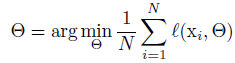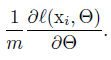• mini-batch的梯度是对整个数据集梯度的估计，当数据集过大时，计算量暴增，使用mini-batch能提高梯度更新的效率（可参考GoodFellow的DeepLearning教材）
• 对于含有m个单独样本的mini-batch的一次计算比单独计算m次更快，这得益于并行计算。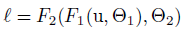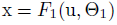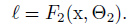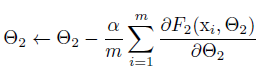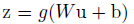# 2 Towards Reducing Internal Covariate Shift

1. 白化的解释可参考 UFLDL的教程4
2. Covariate Shift的解释可以参考这里

# 3 Normalization via Mini-Batch Statistics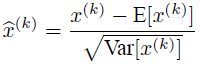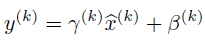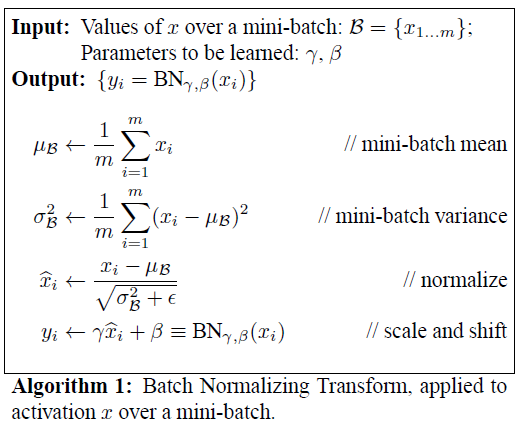BN算法可以添加到网络中来控制激活。注意到BN（）并不是单独地在每一个训练样本中处理激活，而是既依赖训练样本，也依赖于mini-batch中的其他样本。经过伸缩和平移变换的y$y$ 传播到其他层。如果忽略ε$\varepsilon$ ，只要每一个mini-batch的元素是从同一个分布中采样，x^$\hat{x}$ 的取值分布都满足零均值和单位方差。子网络的输入具有固定的均值和方差。虽然所有x^(k)$\hat{x}^{(k)}$ 的联合分布会在训练过程中发生改变。由于标准化子网络的引入会加速子网络的训练过程，最终加速整个网络的训练过程。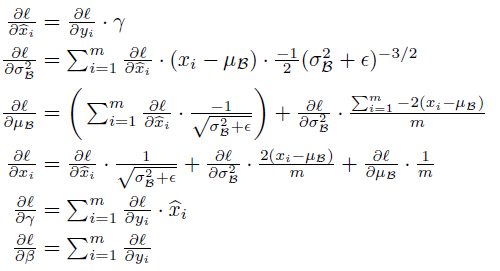## 3.1 Training and Inference with Batch-Normalized Networks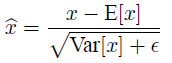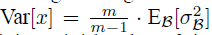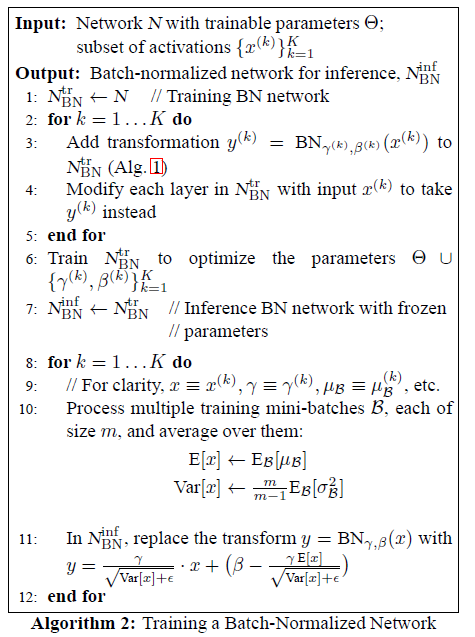## 3.2 Batch-Normalized Convolutional Networks

BN可以被应用到任何网络的激活中。考虑一个如下的变换：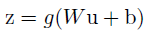z=g(BN(Wu))$z=g(BN(Wu))$
BN变换是应用到x=Wu$x=Wu$ 的每一个维度。在每一个维度都具有一对独立的参数γ(k)$\gamma^{(k)}$β(k)$\beta^{(k)}$

## 3.3 Batch Normalization enables higher learning rates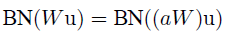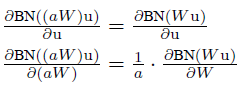z^=F(x^)$\hat{z}=F(\hat{x})$

F(x^)Jx^$F(\hat{x})\approx J \hat{x}$ 对于给定的模型参数就近似于一个线性变换。并且如果z^$\hat{z}$x^$\hat{x}$ 都具有单位协方差，即I=cov[z^]=Jcov[x^]JT=JJT$I = cov[\hat{z}]=Jcov[\hat{x}]J^{T}=JJ^{T}$，因此J$J$ 的所有奇异值为1，这在反向啊传播的过程中可以稳定梯度的量级。实际的变换是非线性的，标准化之后的输入也不可能完全保证正态分布或者完全独立，但是BN仍然可以使反向传播的过程表现得更好。

# 4 Experiments

## 4.1 Activations over time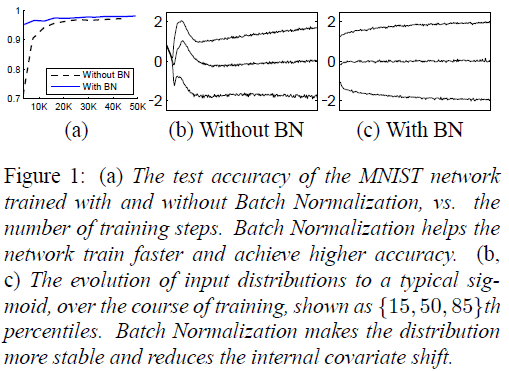## 4.2 ImageNet classification

• 增大学习率
• 去除Dropout层
• 减少L2正则
• 增大学习率衰减
• 去除LRN
• 更加彻底地打乱训练样本
• 减少图像亮度失真

### 4.2.2 Single-Network Classification

• Inception：学习率0.0015
• BN-Baseline：Inception+BN
• BN-x5：Inception+BN+4.2.1中的改进，学习率0.0075
• BN-x30：与BN-x5类似，初始学习率为0.045
• BN-x5-Sigmoid：与BN-x5类似，但是使用sigmoid函数而不是ReLU
Figure 2给出了验证集上整个训练过程中的正确率，可以看出，达到Inception相同正确率的水平，BN-x5训练步数最少。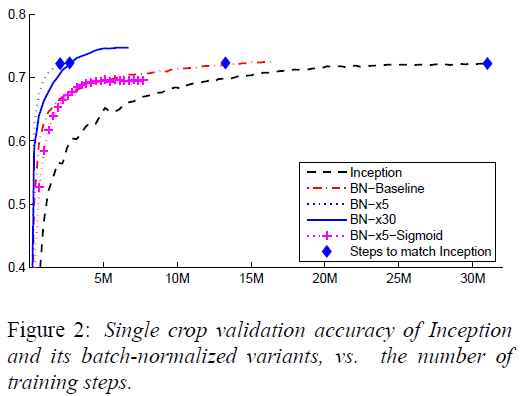Figure 3给出了最大正确率和训练步数的表格：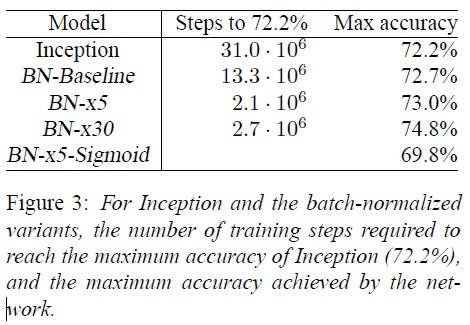# 5 Conclusion

input_shape = self.input_shape
reduction_axes = list(range(len(input_shape)))
del reduction_axes[self.axis]
broadcast_shape =  * len(input_shape)  #len()用来统计行数
broadcast_shape[self.axis] = input_shape[self.axis]
if train:
m = K.mean(X, axis=reduction_axes)  #求各维度均值
brodcast_m = K.reshape(m, broadcast_shape)  #展开均值
std = K.mean(K.square(X - brodcast_m) + self.epsilon, axis=reduction_axes)   #求方差
std = K.sqrt(std)  #求标准差
brodcast_std = K.reshape(std, broadcast_shape)  #展开标准差
mean_update = self.momentum * self.running_mean + (1-self.momentum) * m  #更新均值
std_update = self.momentum * self.running_std + (1-self.momentum) * std  #更新方差
self.updates = [(self.running_mean, mean_update),
(self.running_std, std_update)]
X_normed = (X - brodcast_m) / (brodcast_std + self.epsilon)  #标准化
else:
brodcast_m = K.reshape(self.running_mean, broadcast_shape)
brodcast_std = K.reshape(self.running_std, broadcast_shape)
X_normed = ((X - brodcast_m) /
(brodcast_std + self.epsilon))
out = K.reshape(self.gamma, broadcast_shape) * X_normed + K.reshape(self.beta, broadcast_shape) 

1. Improving predictive inference under covariate shift by weighting the log-likelihood function
2. LeCun, Y., Bottou, L., Orr, G., and Muller, K. Efficient
backprop. In Orr, G. and K., Muller (eds.), Neural Networks:
Tricks of the trade. Springer, 1998b.
3. Wiesler, Simon and Ney, Hermann. A convergence analysis
of log-linear training. In Shawe-Taylor, J., Zemel,
R.S., Bartlett, P., Pereira, F.C.N., andWeinberger, K.Q.
(eds.), Advances in Neural Information Processing Systems
24, pp. 657–665,Granada, Spain, December 2011.
4. http://ufldl.stanford.edu/wiki/index.php/%E7%99%BD%E5%8C%96
5. LeCun, Y., Bottou, L., Orr, G., and Muller, K. Efficient
backprop. In Orr, G. and K., Muller (eds.), Neural Networks:
Tricks of the trade. Springer, 1998b.
6. Saxe, Andrew M., McClelland, James L., and Ganguli,
Surya. Exact solutions to the nonlinear dynamics
of learning in deep linear neural networks. CoRR,
abs/1312.6120, 2013.